# SSD-VGG-300 Trained onPASCAL VOC Data

Contributed By: Julian W. Francis

Detect and localize objects in an image

Released in 2016, this model discretizes the output space of bounding boxes into a set of default boxes. At the time of prediction, scores are generated for each object and multiple feature maps with different resolutions are used to make predictions for objects of various sizes. This model processes images at 59 FPS on a NVIDIA Titan X.

Number of layers: 145 | Parameter count: 27,076,694 | Trained size: 109 MB |

## Examples

### Resource retrieval

Get the pre-trained net:

 In:=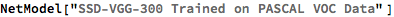Out=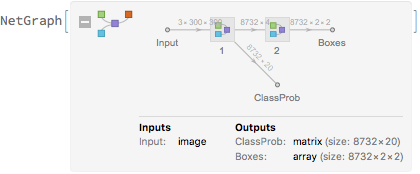### Evaluation function

Write an evaluation function to scale the result to the input image size and suppress the least probable detections:

 In:=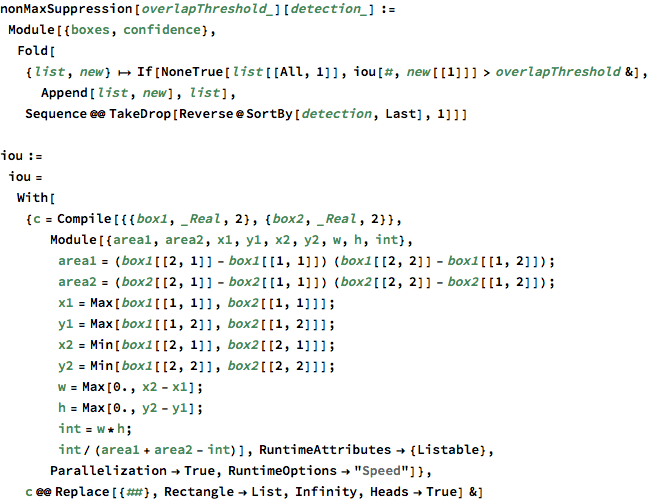Define the label list for this model. Integers in the model's output correspond to elements in the label list:

 In:=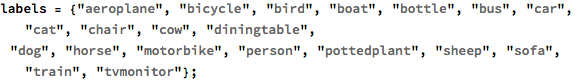In:=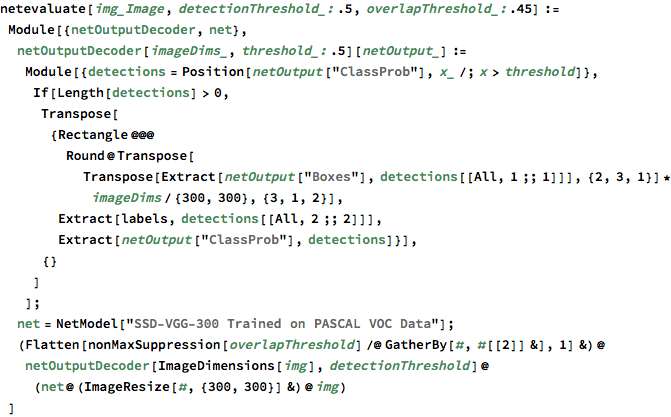### Basic usage

Obtain the detected bounding boxes with their corresponding classes and confidences for a given image:

 In:=In:=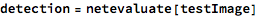Out=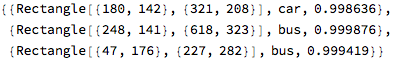Inspect which classes are detected:

 In:=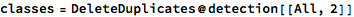Out=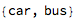Visualize the detection:

 In:=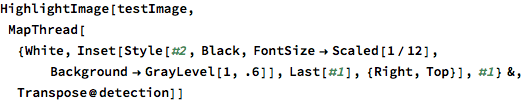Out=### Network result

The network computes 8,732 bounding boxes and the probability that the objects in each box are of any given class:

 In:=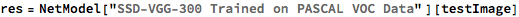Out=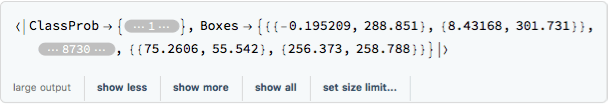Visualize all the boxes predicted by the net scaled by their “objectness” measures:

 In:=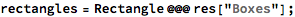In:=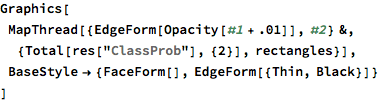Out=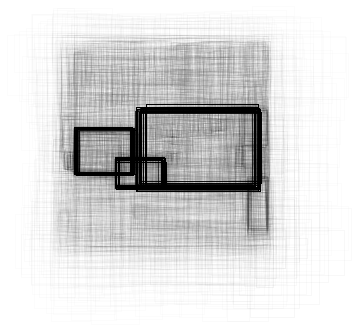Visualize all the boxes scaled by the probability that they contain a bus:

 In:=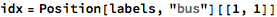Out=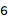In:=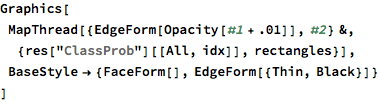Out=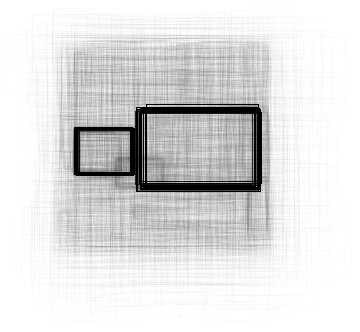Superimpose the bus prediction on top of the scaled input received by the net:

 In:=Out=Write a function to apply a custom styling to the result of the detection:

 In:=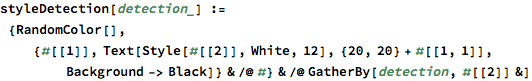Visualize multiple objects, using a different color for each class:

 In:=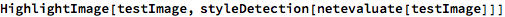Out=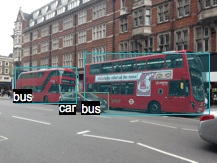### Net information

Inspect the number of parameters of all arrays in the net:

 In:=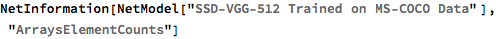Out=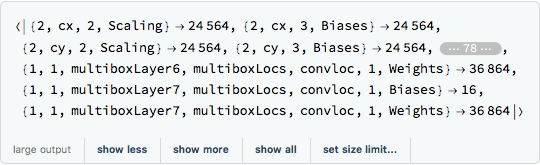Obtain the total number of parameters:

 In:=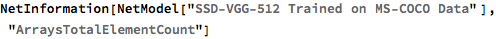Out=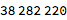Obtain the layer type counts:

 In:=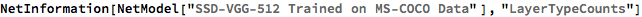Out=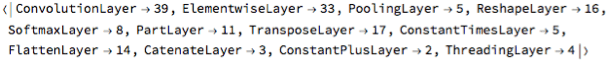Display the summary graphic:

 In:=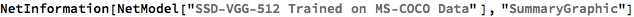Out=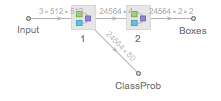### Export to MXNet

Export the net into a format that can be opened in MXNet:

 In:=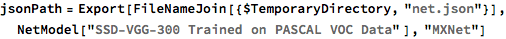Out=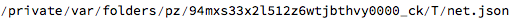Export also creates a net.params file containing parameters:

 In:=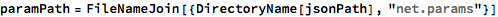Out=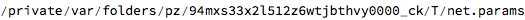Get the size of the parameter file:

 In:=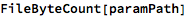Out=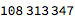The size is similar to the byte count of the resource object:

 In:=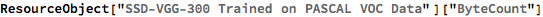Out=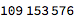## Requirements

Wolfram Language 11.3 (March 2018) or above